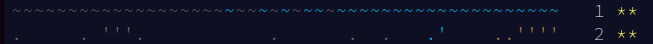# Advent Of Code 2021 – Dive! – Puzzle 2

Hello ! I’m Xavier Jouvenot and here is the 2nd part of a long series on Advent Of Code 2021.

For this new post, we are going to solve the problem from the 2nd December 2021, named "Dive!". The solution I will propose in C++, but the reasoning can be applied to other languages.

Self promotion: You can find other articles on computer science and programming on my website 😉

## Part 1

### The Problem

The full version of this problem can be found directly on the Advent of Code website, I will only describe the essence of the problem here:

After using the sonar to identify our surroundings, during the Day 1, we now want to move with our submarine. In order to do so, the submarine can understand 3 kind of instructions:

• `forward X` increases the `horizontal position` by X units.
• `down X` increases the `depth` by X units.
• `up X` decreases the `depth` by X units.

Since the submarine already has a sets of instruction programmed, we need to know where we are going, and to figure out at which `depth` and `horizontal position` we are going to end up. To have only one answer to give to the problem (and to the website), we have to multiply the 2 values we are going to found and the result will be our answer.

### Solution

So, we are going to need to be able to do 2 things:

• Identify which instruction is given to us
• Modify either the `depth` or `horizontal position` accordingly to the instruction we read

In C++, it looks like that:

```#include <array>
#include <cassert>
#include <iostream>
#include <string>
#include <string_view>

constexpr std::array<std::string_view, 1000> input = { /* ... */ };

constexpr bool isForwardInstruction (const std::string_view instruction) { return instruction.substr(0, 7) == "forward" ;}
constexpr bool isDownInstruction (const std::string_view instruction) { return instruction.substr(0, 4) == "down" ;}
constexpr bool isUpInstruction (const std::string_view instruction) { return instruction.substr(0, 2) == "up" ;}

int main()
{
size_t horizontalPosition{0}, depth{0};

for(const auto& instruction : input)
{
if(isForwardInstruction(instruction)) { horizontalPosition += std::stoi(instruction.substr(8).data()); continue; }
if(isDownInstruction(instruction)) { depth += std::stoi(instruction.substr(5).data()); continue; }
if(isUpInstruction(instruction)) { depth -= std::stoi(instruction.substr(3).data()); continue; }
assert(false); // Unkwnow input
}

std::cout << "The solution is: " << (horizontalPosition * depth) << std::endl;

return 0;
}
```

As you can see, the first step, is handled by the 3 functions `isForwardInstruction`, `isDownInstruction` and `isUpInstruction` which allow to identify the current instruction that we are reading.

And in the for loop, which go through the instructions, we modify the corresponding value accordingly to the definition of the instruction in the problem: the `substr` function call allows us to extract the number related to the instruction, as a string of character, and the std::stoi function call transforms the string character into an actual number that we can use to add or subtract to the variables.

Finally, we display the result wanted by multiplying the 2 variables containing the information we have extracted from the list of instructions.

## Part 2

### Problem

Apparently the previous method of calculation was just wrong 😛

So, we now need to track another variable: the `aim` ! And the instruction must be interpreted as follow:

• down X increases your aim by X units.
• up X decreases your aim by X units.
• forward X does two things:
• It increases your horizontal position by X units.

Even if we have a new variable, the final result will still be the result of the multiplication of the `depth` and the `horizontal position` of the submarine. 😉

### Solution

Well the code, for the solution of the part 1, is only a little bit changed actually ! All we have to do, is to change the code related to each instruction, meaning that the code of the for loop is now:

```if(isDownInstruction(instruction)) { aim += std::stoi(instruction.substr(5).data()); continue; }
if(isUpInstruction(instruction)) { aim -= std::stoi(instruction.substr(3).data()); continue; }
if(isForwardInstruction(instruction))
{
const auto units = std::stoi(instruction.substr(8).data());
horizontalPosition += units;
depth += units * aim;
continue;
}
```

As the problem specified, in the 2 first instructions, the variable `aim` is modified, and in the last one, we modify the `depth` and the `horizontal position` taking the `aim` into account.

If you want, you can look at the full code in the file versioned on GitHub 😉